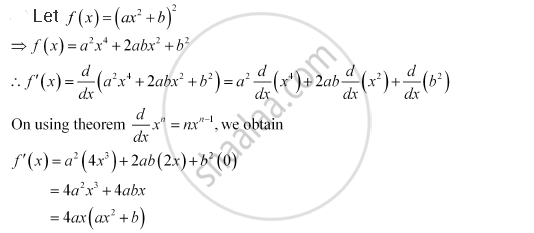CBSE (Commerce) Class 11CBSE
Share
Notifications

View all notifications
Books Shortlist
Your shortlist is empty

# Solution for For Some Constants A And B, Find the Derivative Of (Ax2 + B)2 - CBSE (Commerce) Class 11 - Mathematics

Login
Create free account

Forgot password?
ConceptDerivative Derivative of Polynomials and Trigonometric Functions

#### Question

For some constants a and b, find the derivative of (ax2 + b)2

#### SolutionIs there an error in this question or solution?

#### APPEARS IN

NCERT Solution for Mathematics Textbook for Class 11 (2013 to Current)
Chapter 13: Limits and Derivatives
Q: 7.2 | Page no. 313
Solution For Some Constants A And B, Find the Derivative Of (Ax2 + B)2 Concept: Derivative - Derivative of Polynomials and Trigonometric Functions.
S